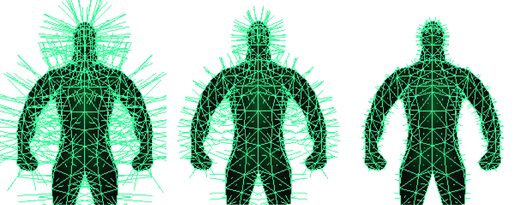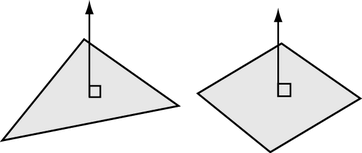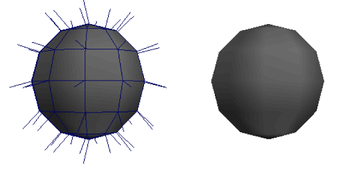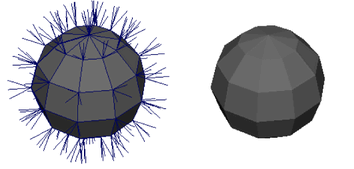# Polygon normals

A normal is a theoretical line that is perpendicular to the surface of a polygon. In Maya, normals are used to determine the orientation of a polygon face (face normals), or how the edges of faces will visually appear in relation to each other when shaded (vertex normals).## Face normals

The front of a polygon’s face is graphically represented using a vector called the polygon’s normal. A normal is a theoretical line representing the direction perpendicular to a polygonal surface.The order of vertices around the face determine the direction of the face (whether a side of the polygon is the front or the back). This can be important because technically polygons are only visible from the front, though by default Maya automatically makes all polygons double-sided so you can see them from the back. You can turn this double-sided behavior off for meshes.

When you shade or render polygons, the normals determine how light reflects from the surface and the shading that results.

## Vertex normals

Vertex normals determine the visual smoothing between polygon faces. Unlike face normals, they are not intrinsic to the polygon, but rather reflect how Maya renders the polygons in smooth shaded mode.

Vertex normals appear as lines projecting from the vertex, one for each face that shares the vertex.

• When the vertex normals at a particular point on the mesh all point in the same direction (called soft or shared vertex normals), there is a soft edge transition between the faces in smooth shaded mode.• When the vertex normals point in the same directions as their faces (called hard vertex normals), the transition between faces is hard, creating a faceted appearance.Advanced users may want to manually manipulate vertex normals to create the appearance of hard edges (creases) and shadows without using additional geometry. You can manually manipulate the vertex normals usingNormals > Vertex Normal Edit Tool. When the vertex normals for a polygon mesh are manually edited they become locked or frozen. When you unlock a previously locked vertex normal, Maya automatically recalculates the vertex normal for the face based on its default normal calculations.

### Related topicsExcept where otherwise noted, this work is licensed under a Creative Commons Attribution-NonCommercial-ShareAlike 3.0 Unported License